• 深度学习——时间序列模型评价指标总结
千次阅读
2021-09-08 07:53:59

大Y老师：小X，你做的这个时间序列模型用什么指标评价啊？

小X 直接把这篇甩给老师：老师您看哪个指标顺眼，就选哪个指标~

最近在实现基于深度学习的时间序列模型时，需要对模型进行评价，总结了一些常用的和不常用的评价指标，还有相关概念的辨析。

上面是玩笑话，在对模型进行评价时，要根据实际的数据特征和指标特性进行选择。

##### 和方差

SSE（the sum of squares due to error），是观测值（observed values）与预测值（predicted values）的误差的平方和，公式为：
S S E ( y , y ^ ) = ∑ i = 1 n ( y i − y ^ i ) 2 SSE(y,\widehat{y})=\sum_{i=1}^{n}(y_{i}-\widehat{y}_{i})^{2}

##### 均方误差

MSE（mean squared error），是观测值（observed values）与预测值（predicted values）的误差的平方和的均值，即SSE/n。它是误差的二阶矩，包含估计量的方差（variance）及其偏差（bias），是衡量估计量质量的指标，其公式为：
M S E ( y , y ^ ) = 1 n ∑ i = 1 n ( y i − y ^ i ) 2 MSE(y,\widehat{y})=\tfrac{1}{n}\sum_{i=1}^{n}(y_{i}-\widehat{y}_{i})^{2}

##### 均方根误差

RMSE（root mean squared error），也称作RMSD(root mean square deviation)，是MSE的算数平方根。由于每个误差（each error）对RMSD的影响与误差的平方（squared error）成正比，因此较大的误差会对RMSE影响过大，RMSE对异常值很敏感。其公式为：
R M S E ( y , y ^ ) = 1 n ∑ i = 1 n ( y i − y ^ i ) 2 RMSE(y,\widehat{y})=\sqrt{\tfrac{1}{n}\sum_{i=1}^{n}(y_{i}-\widehat{y}_{i})^{2}}

##### 平均绝对值误差

MAE（mean absolute error），是时间序列分析中预测误差常用的指标，由于MAE使用的是与被测数据相同的尺度（scale）,因此不能用于比较两个不同尺度的序列。MAE又被称为L1范数损失函数（就是可以做为损失函数），是真实数据与预测数据之差的绝对值的均值。

公式为：
M A E ( y , y ^ ) = 1 n ∑ i = 1 n ∣ y i − y ^ i ∣ MAE(y,\widehat{y})=\tfrac{1}{n}\sum_{i=1}^{n}\left | y_{i}-\widehat{y}_{i}\right |

##### 平均绝对值百分比误差

MAPE（mean absolute percentage error），也被称为MAPD（mean absolute percentage deviation），是一种衡量预测方法的预测准确性的指标。MAPE在解释相对误差（relative error）方面非常直观，在评价模型时MAPE通常用作回归（regression）问题的损失函数（loss function）。

从式子中可以看出，在计算MAPE时如果出现一系列特别小的分母，可能会出现一些问题。比如分母为0的奇异点、较小的误差引起结果发生非常大的变化等。

解决这个问题的替代方案，可以将公式中的实际值，替换为该序列的所有实际值的平均值。这种方案等效于求绝对差的总和除以实际值的总和，也被称为加权绝对百分比误差（WAPE）,或者wMAPE（weighted mean absolute percentage error）。

公式为：
M A P E ( y , y ^ ) = 100 % n ∑ i = 1 n ∣ y i − y ^ i y i ∣ MAPE(y,\widehat{y})=\tfrac{100\% \displaystyle }{n}\sum_{i=1}^{n}\left | \frac{y_{i}-\widehat{y}_{i}}{y_{i}}\right |

##### 对称平均绝对百分比误差

SMAPE（symmetric mean absolute percentage error），实际值与预测值差值的绝对值除以实际值与预测值绝对值之和的一半。公式为：
S M A P E ( y , y ^ ) = 100 % n ∑ i = 1 n ∣ y i − y ^ ∣ ∣ ∣ y i ∣ + ∣ y ^ i ∣ 2 ∣ SMAPE(y,\widehat{y})=\frac{100\%}{n}\sum_{i=1}^{n}\frac{\left | y_{i}-\widehat{y}\right |}{\left | \frac{\left |y_{i} \right | + \left |\widehat{y}_{i}\right |}{2}\right |}

##### 均方对数误差

MSLE（mean squared log error），公式为：

M S L E ( y , y ^ ) = 1 n ∑ i = 1 n ( l o g ( 1 + y i ) − l o g ( 1 + y ^ i ) ) 2 MSLE(y,\widehat{y})=\frac{1}{n}\sum_{i=1}^{n}\left ( log(1+y_{i})-log(1+\widehat{y}_{i})\right )^{2}

##### 绝对中位差

公式为：

M A D ( y , y ^ ) = m e d i a n ( ∣ y 1 − y ^ 1 ∣ , . . . , ∣ y n − y ^ n ∣ ) MAD(y,\widehat{y})= median(\left | y_{1} - \widehat{y}_{1}\right |,...,\left | y_{n} - \widehat{y}_{n}\right |)
论文中常用的指标：

##### 结构相似性

SSMI（structural similarity ）,用于衡量两幅图像的相似度，公式为：
S S I M ( x , y ) = ( 2 μ x μ y + C 1 ) ( 2 σ x y + C 2 ) ( μ x 2 + μ y 2 + C 1 ) ( σ x 2 + σ y 2 + C 2 ) SSIM(x,y)= \frac{(2\mu_{x}\mu_{y} + C_{1})(2\sigma_{xy} + C_{2})}{(\mu_{x}^{2}+\mu_{y}^{2}+C_{1})(\sigma _{x}^{2}+\sigma _{y}^{2}+C_{2})}
其中x为预测图像的像素值，y为真实图像的像素值。𝜇𝑥为𝑥的均值，𝜇𝑦为𝑦的均值，𝜎𝑥 2为𝑥的方差，𝜎𝑦 2为𝑦的方 差， 𝜎𝑥𝑦为𝑥和𝑦的协方差，𝐶1 、𝐶2 是像素值的动态范围。SSIM的范围为0~1，值越大说明两幅图像越相似。

##### 临界成功指数

CSI（critical success index），公式为：
C S I = a a + b + c CSI=\frac{a}{a+b+c}

##### 命中率

POD（probability of detection），公式为：
P O D = a a + c POD=\frac{a}{a+c}

##### 虚警率

FAR（false alarm rate），公式为：
F A R = b a + b FAR=\frac{b}{a+b}
其中：

a为命中数，即预测值与实际值均大于判别阈值的数量。

b为空报数，即预测值大于判别阈值而实际值小于判别阈值。

c为漏报数，即预测值小于判别阈值而真实值大于判别阈值。

##### 方差

Variance，用于衡量随机变量离散程度的度量，是各样本数据与平均值之差的平方和的均值。公式为：
V a r = 1 n ∑ i = 1 n ( x i − μ ) 2 Var=\frac{1}{n}\sum_{i=1}^{n}\left ( x_{i}-\mu\right )^{2}
其中，xi为变量，𝜇为总体均值，n为样本总数。

##### 标准差

SD（standard deviation），又称均方差，是方差的平方根，公式为：
S D = 1 n ∑ i = 1 n ( x i − μ ) 2 SD= \sqrt{\frac{1}{n}\sum_{i=1}^{n}\left ( x_{i}-\mu\right )^{2}}

##### 方差、标准差、均方误差、均方根误差辨析：

1.标准差就是均方差，不是均方误差。

2.方差与均方误差形式上接近，但概念不同。方差是样本数据与样本平均值差值的平方和的均值。均方误差是预测数据与真实数据差值的平方和的均值。

3.同样，标准差与均方根误差形式上接近，但概念不同。深度学习 机器学习
更多相关内容
• 关于时间序列模型的比较准确的描述，可以通过这个pdf来学习一下传统的经典时间序列模型
• 模糊时间序列模型和季节模型都是基于时间序列的模型，为了探讨在时间序列表现出一定的周期性时，哪种模型的预测效果会更好，分别利用模糊时间序列模型和季节模型对南京某商场的客流量进行预测，计算并比较两种方法下...
• 时间序列预测建模，移动平滑、指数平滑、等模型的描述讲解和matlab程序实现代码。arima、arma等等
• 把时间作为自变量，相应序列观察值作为因变量，简历回归模型 组合模型法 受长期趋势（T）、季节变动（S）、周期变动（C）和不规则变动（ε）四个因素影响 组合模型 （1）加法模型：T+S+C+ϵT+S+C+\epsilonT+S+C+...

模型名称描述
平滑法削弱短期随机波动对序列的影响，序列插值分布均匀
趋势拟合法把时间作为自变量，相应序列观察值作为因变量，简历回归模型
组合模型法受长期趋势（T）、季节变动（S）、周期变动（C）和不规则变动（ε）四个因素影响
组合模型（1）加法模型： T + S + C + ϵ T+S+C+\epsilon （2）乘法模型： T S C ϵ T SC\epsilon

常见时间序列模型

模型名称描述
AR模型考虑历史数据的影响，以过往数据为自变量， X t X_t 为因变量建立线性回归模型。 X t = ϕ 0 + ϕ 1 x t − 1 + ⋯ + ϕ p x t − p X_t=\phi_0+\phi_1 x_{t-1}+\cdots+\phi_p x_{t-p}
MA模型忽略历史数据影响，建立于前q期随机扰动 ϵ t − 1 , ⋯   , ϵ t − q \epsilon_{t-1},\cdots,\epsilon_{t-q} 的线性回归模型 X t = μ + ϵ t − θ 1 ϵ t − 1 − ⋯ − θ q ϵ t − q X_t=\mu+\epsilon_t-\theta_1 \epsilon_{t-1}-\cdots-\theta_q \epsilon_{t-q}
ARMA模型结合考虑AR、MA模型，综合考虑它们的影响
ARIMA模型针对非平稳序列。将非平稳转化为平稳后拟合操作

# 1. 基本概念

白噪声序列： 数据随机分布，没有规律。
平稳非白噪声序列
非平稳序列： 可以利用差分法转换为平稳非白噪声序列
截尾： 拖尾指序列以指数率单调递减或震荡衰减
拖尾： 截尾指序列从某个时点变得非常小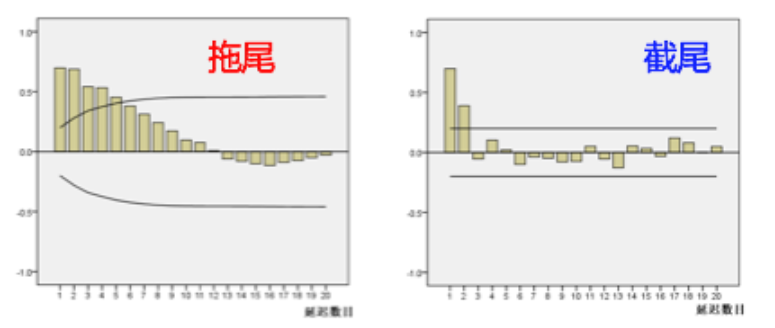## 1.1 自相关函数ACF（autocorrelation function）

• 自相关函数反映了同一序列在不同时序的取值之间的相关性。
• 公式：
A C F ( k ) = ρ k = C o v ( y t , y t − k ) V a r ( y t ) ACF(k) = \rho_k=\frac{Cov(y_t,y_{t-k})}{Var(y_t)}
其中， ρ k ∈ [ − 1 , 1 ] \rho_k \in[-1,1]

## 1.2 偏自相关函数PACF（partial autocorrelation function）

• 剔除了中间k-1个随机变量 x ( t − 1 ) , x ( t − 2 ) , ⋯   , x ( t − k + 1 ) x(t-1),x(t-2),\cdots,x(t-k+1) 的干扰后 x ( t − k ) x(t-k) x ( t ) x(t) 影响的相关程度
• PACF是严格两个变量之间的相关性。

# 2. 常见模型

## 2.1 自回归模型（AR）

定义：

• 描述当前值与历史值之间的关系。利用历史数据对自身进行预测

• P阶自回归过程，即当前值与 t − p , t − p + 1 , ⋯   , t − 1 t-p,t-p+1,\cdots,t-1 相关（P）。
y t = μ + ∑ i = 1 p γ i y t − i + ϵ t y_t=\mu+\sum_{i=1}^{p} \gamma_i y_{t-i} + \epsilon_t
其中， y t y_t 是当前值， μ \mu 是常数项，P是阶数， γ i \gamma_i 是自相关系数， ϵ \epsilon 是误差。

• 参数：P（自回归阶数）

注意

• 必须具有平稳性
• 必须具有自相关性，自相关系数需要大于等于0.5.

模型识别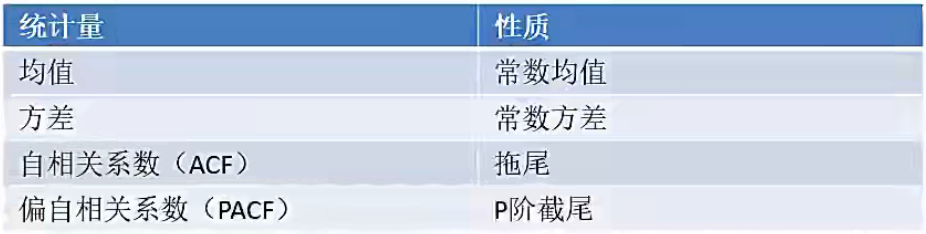## 2.2 移动平均模型（MA）

定义

• 移动平均模型是自回归模型中误差项的累加

• q阶移动模型公式（Q）：
y t = μ + ϵ t + ∑ i = 1 q θ i ϵ t − i y_t = \mu + \epsilon_t + \sum_{i=1}^{q} \theta_i \epsilon_{t-i}

• 移动平均法能有效地消除预测中的随机波动

• 参数：Q（移动平均阶数）

模型识别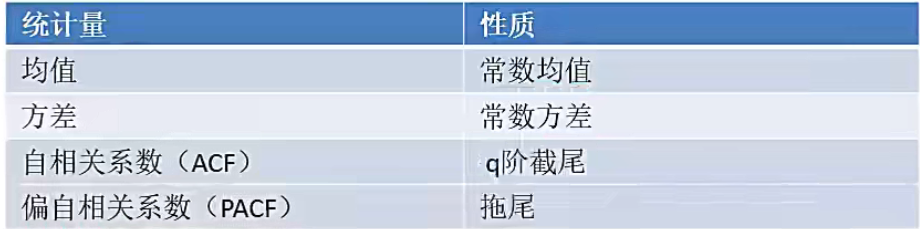## 2.3 自回归移动平均模型（ARMA）

定义

• 自回归与移动平均的结合

• 公式定义为（P,Q）：
y t = μ + ∑ i = 1 p γ i y t − i + ϵ t + ∑ i = 1 q θ i ϵ t − i y_t = \mu + \sum_{i=1}^{p} \gamma_i y_{t-i} + \epsilon_t + \sum_{i=1}^{q} \theta_i \epsilon_{t-i}

• 参数：P（自回归阶数），Q（移动平均阶数）

模型识别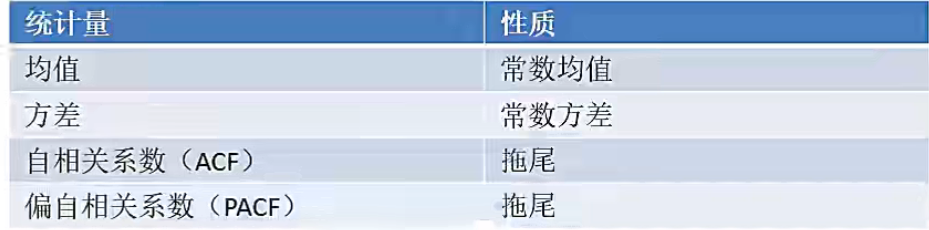## 2.4 差分自回归移动平均模型（ARIMA）

对于ARIMA模型，我们需要指定三个参数（P, D, Q），分别表示P阶自回归模型，D阶差分和Q阶移动平均模型。
定义：

• 参数：（P, D, Q），分别表示P阶自回归模型，D阶差分和Q阶移动平均模型。
• 原理：将非平稳时间序列转换为平稳时间序列，然后将因变量对其滞后值和其随机误差的滞后值进行回归建模。

# 4. 建模步骤

## 4.1 平稳性检验

### 4.1.1 时序图、自相关图、偏自相关图

def tsplot(y,lags=None,figsize=(12,7),style='bmh'):
'''
Plot time series, its ACF and PACF, calculate Dickey-Fuller test
y:timeseries
lags:how many lags to include in ACF,PACF calculation
'''
#     if not isinstance(y, pd.Series):
#         y = pd.Series(y)
with plt.style.context(style):
fig = plt.figure(figsize=figsize)
layout=(2,2)
ts_ax = plt.subplot2grid(layout, (0,0), colspan=2)
acf_ax = plt.subplot2grid(layout, (1,0))
pacf_ax = plt.subplot2grid(layout, (1,1))

y.plot(ax=ts_ax)
ts_ax.set_title('Time Series Analysis Plots\n Dickey-Fuller: p={0:.5f}'.format(p_value))
smt.graphics.plot_acf(y,lags=lags, ax=acf_ax)
smt.graphics.plot_pacf(y,lags=lags, ax=pacf_ax)
plt.tight_layout()
tsplot(data)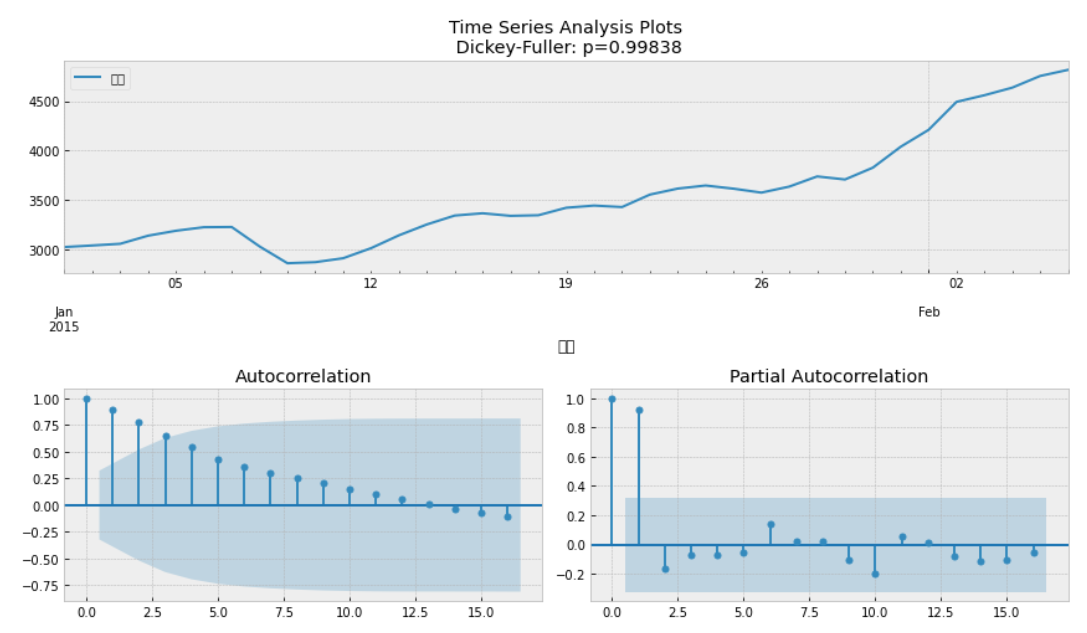### 4.1.2 自相关、偏自相关图

根据自相关图，判断“拖尾”、“截尾”。

from statsmodels.graphics.tsaplots import plot_acf
plot_acf(data).show()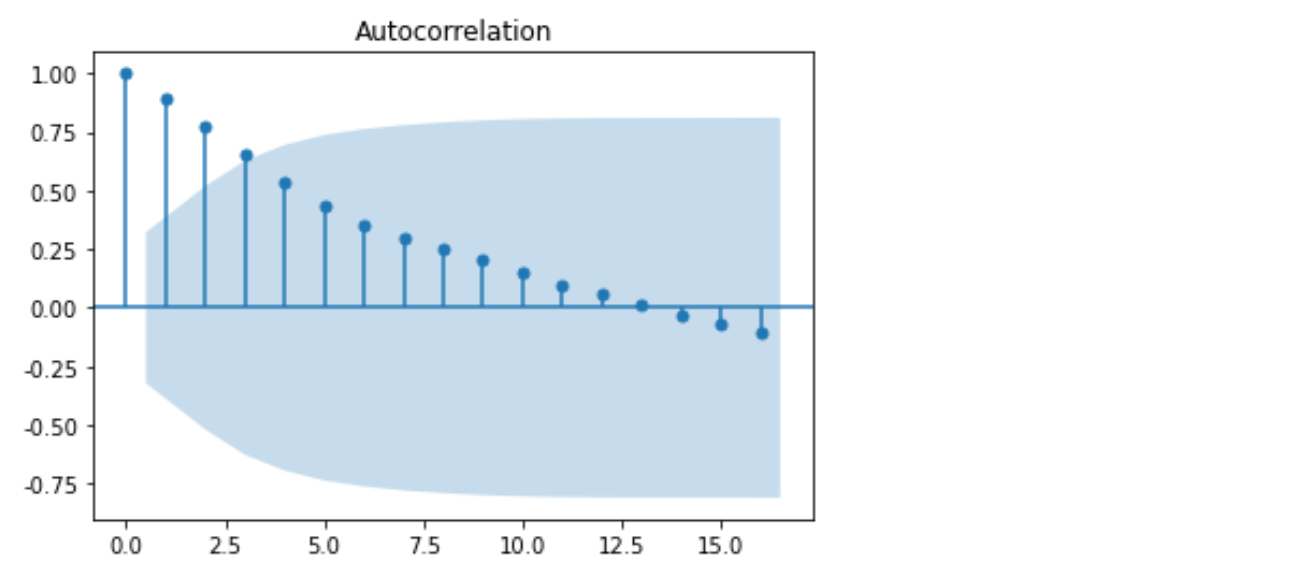from statsmodels.graphics.tsaplots import plot_pacf
plot_pacf(data).show()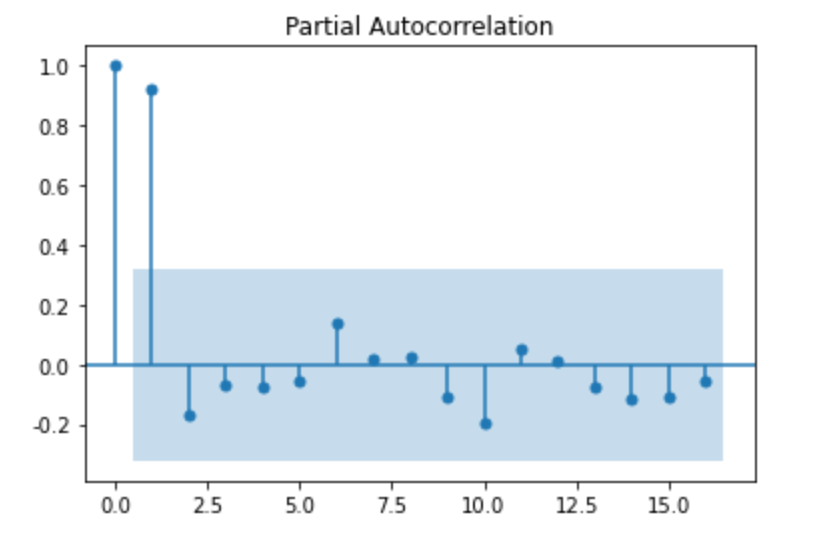### 4.1.3 单位根检验

采用单位根法检验，当单位根大于等于0.05时，表示数据为非平稳序列。

from statsmodels.tsa.stattools import adfuller as ADF


(1.813771015094526, 0.9983759421514264, 10, 26, {‘1%’: -3.7112123008648155, ‘5%’: -2.981246804733728, ‘10%’: -2.6300945562130176}, 299.4698986602418)

### 4.1.4 差分运算

若序列为非平稳序列，则需要转换为平稳序列计算
（1）差分运算

• P阶差分
相距一期的两个序列之间的减法运算称为1阶差分运算
将1阶差分运算的结果再做一次差分运算则称为2阶差分运算
• K步差分
相距k期的两个序列值之间的减法运算称为k步差分运算

1. 差分运算

D_data = data.diff().dropna()    # 一阶一步差分,并去除NA
D_data.columns = ['sale diff']


2. 差分结果检验
对差分结果进行相同的平稳性检验，当单位根值小于等于0.05时，表示已经转换为平稳序列。

# 自相关图
plot_acf(D_data).show()
# 偏自相关图
plot_pacf(D_data).show()
# 单位根值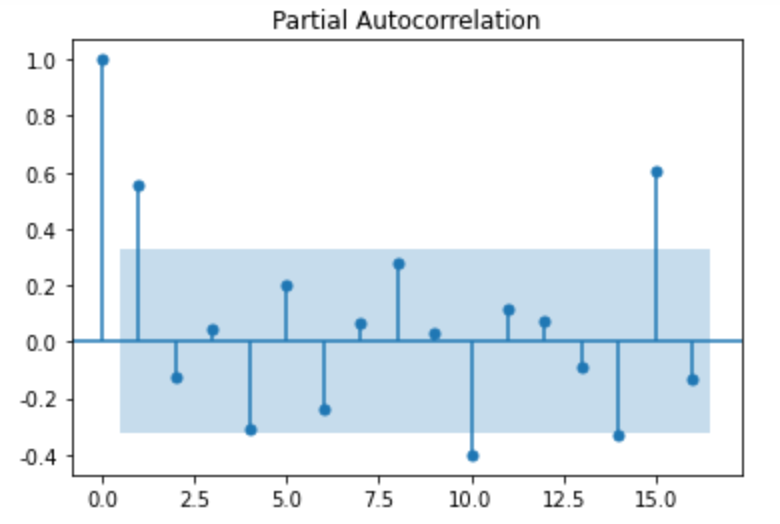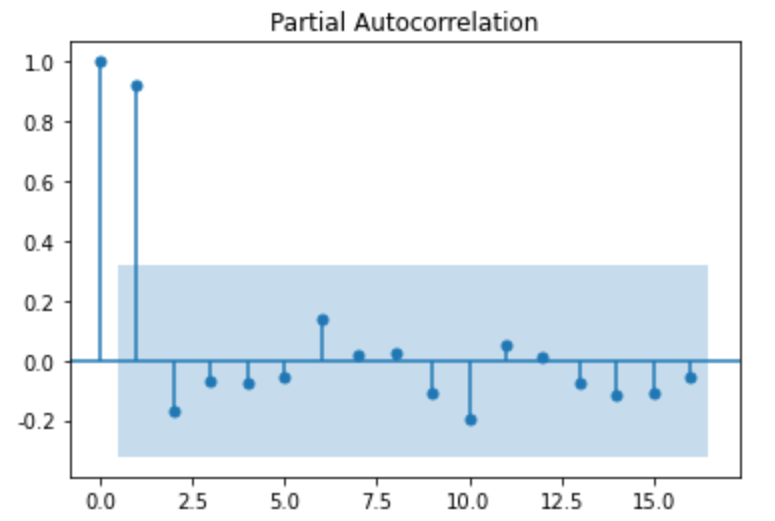(-3.1560562366723537, 0.022673435440048798, 0, 35, {‘1%’: -3.6327426647230316, ‘5%’: -2.9485102040816327, ‘10%’: -2.6130173469387756}, 287.5909090780334)

## 4.2 白噪声检验

from statsmodels.stats.diagnostic import acorr_ljungbox
print('差分序列的白噪声检验结果：')
print(acorr_ljungbox(D_data,lags=1))   # 返回统计量与p值，当p <= 0.05 时，不是白噪音


差分序列的白噪声检验结果：
(array([11.30402222]), array([0.00077339]))

## 4.3 模型选择（p,q,d）

AR, MA模型
通过ACF, PACF截尾开始阶数确定p, q参数值。

• AR§：PACF上截尾，ACF趋近于0
• MA(Q)：ACF上截尾，PACF趋近于0

采用BIC矩阵，找到最小值对应的p, q值。并以此为参数选择下述三个模型：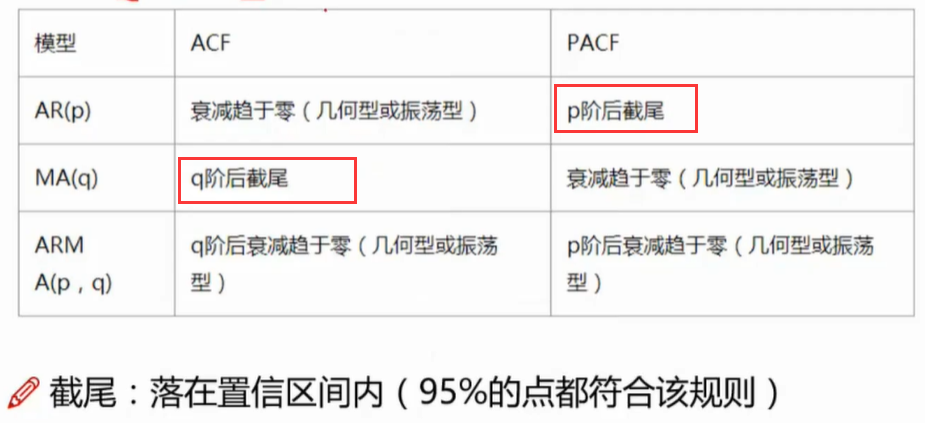### 4.3.1 AIC与BIC

AIC：赤池信息准则（Akaike information Criterion）
A I C = 2 k − 2 ln ⁡ ( L ) AIC = 2k-2\ln(L)

BIC：贝叶斯信息准则（Bayesian information Criterion）
B I C = k ln ⁡ ( n ) − 2 ln ⁡ ( L ) BIC = k\ln(n)-2\ln(L)

其中，k为模型参数个数，n为样本数量，L为似然函数。

• AIC, BIC值越低越好
• 希望通过AIC, BIC选择更简单的模型，P, Q越大，所需参数项数目越多。
from statsmodels.tsa.arima_model import ARIMA
# 定阶
# data['销量']=data['销量'].astype(float)
pmax = int(len(D_data)/10)
qmax = int(len(D_data)/10)

bic_matrix = []          # BIC矩阵
for p in range(pmax+1):
tmp = []
for q in range(qmax+1):
try:    # 部分报错，跳过
tmp.append(ARIMA(data,(p,1,q)).fit().bic)
except:
tmp.append(None)
bic_matrix.append(tmp)

bic_matrix = pd.DataFrame(bic_matrix)
bic_matrix.index = ['AR{}'.format(i) for i in range(pmax+1)]
bic_matrix.columns = ['MA{}'.format(i) for i in range(qmax+1)]
bic_matrix = bic_matrix[bic_matrix.columns].astype(float)

p,q = bic_matrix.stack().idxmin()
print('最小BIC对应的p,q值：%s, %s'%(p,q))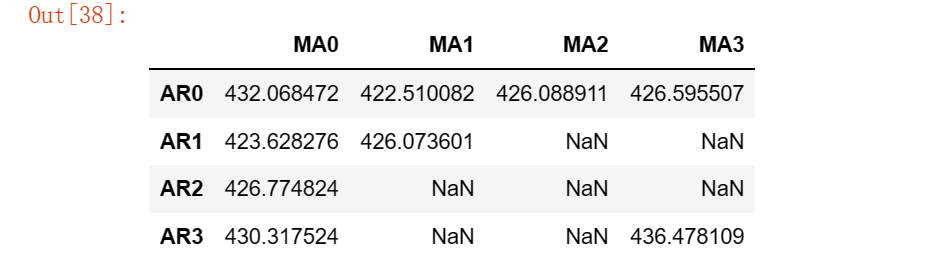最小BIC对应的p,q值：0, 1

import seaborn as sns
fig, ax = plt.subplots(figsize = (10,8))
ax = sns.heatmap(bic_matrix,
ax = ax,
annot = True,
fmt='.2f')
ax.set_title('BIC')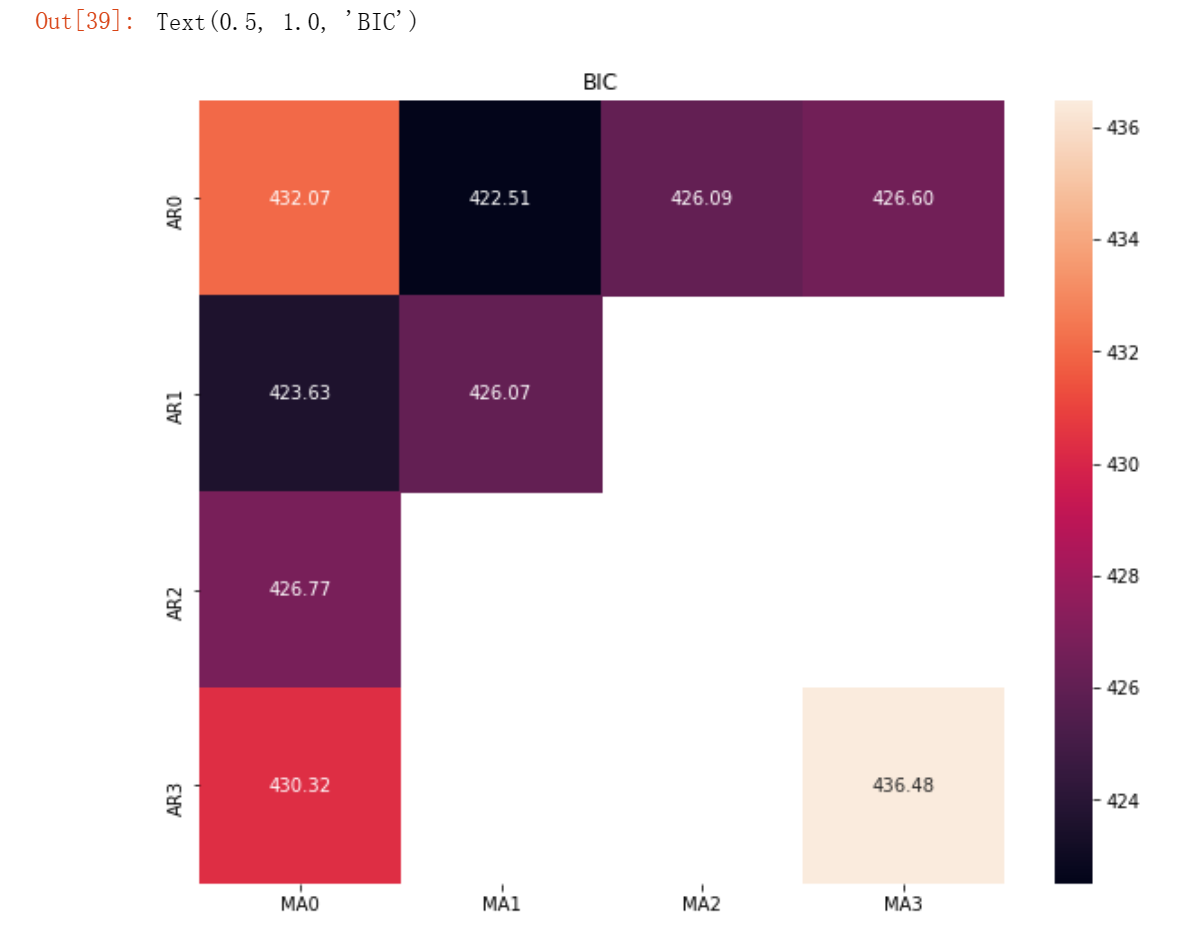result = smt.arma_order_select_ic(data, ic=['aic', 'bic'], trend='nc', max_ar=4, max_ma=4)


{‘aic’: 0 1 2 3 4
0 NaN 668.212060 627.212365 590.498371 565.724666
1 472.121781 442.706792 444.414985 441.613728 442.640421
2 449.878586 443.006993 445.873538 443.364868 445.454087
3 452.992514 441.894814 443.613869 449.670033 442.185777
4 454.442210 443.477934 445.326246 445.745273 445.296295,
‘bic’: 0 1 2 3 4
0 NaN 671.433896 632.045119 596.942043 573.779256
1 475.343616 447.539546 450.858657 449.668317 452.305928
2 454.711339 449.450664 453.928128 453.030375 456.730513
3 459.436186 449.949403 453.279376 460.946459 455.073120
4 462.496800 453.143442 456.602672 458.632617 459.794557,
‘aic_min_order’: (1, 3),
‘bic_min_order’: (1, 1)}

由此可以选择MA模型，或者p =0, q =1的ARMA模型。可以将做完差分的数据带入平稳模型或者将原数据带入非平稳模型，并设置阶数。

### 4.3.2 模型稳定性检验

模型残差检验

• ARIMA的残差是否是平均值为0且方差为常数的正太分布
• QQ图：线性即正太分布

## 4.4 模型预测

model = ARIMA(data, (p,1,q)).fit()
print('模型报告为：\n', model.summary2())
print('未来5天的预测结果、准确误差及置信区间：\n',model.forecast(5))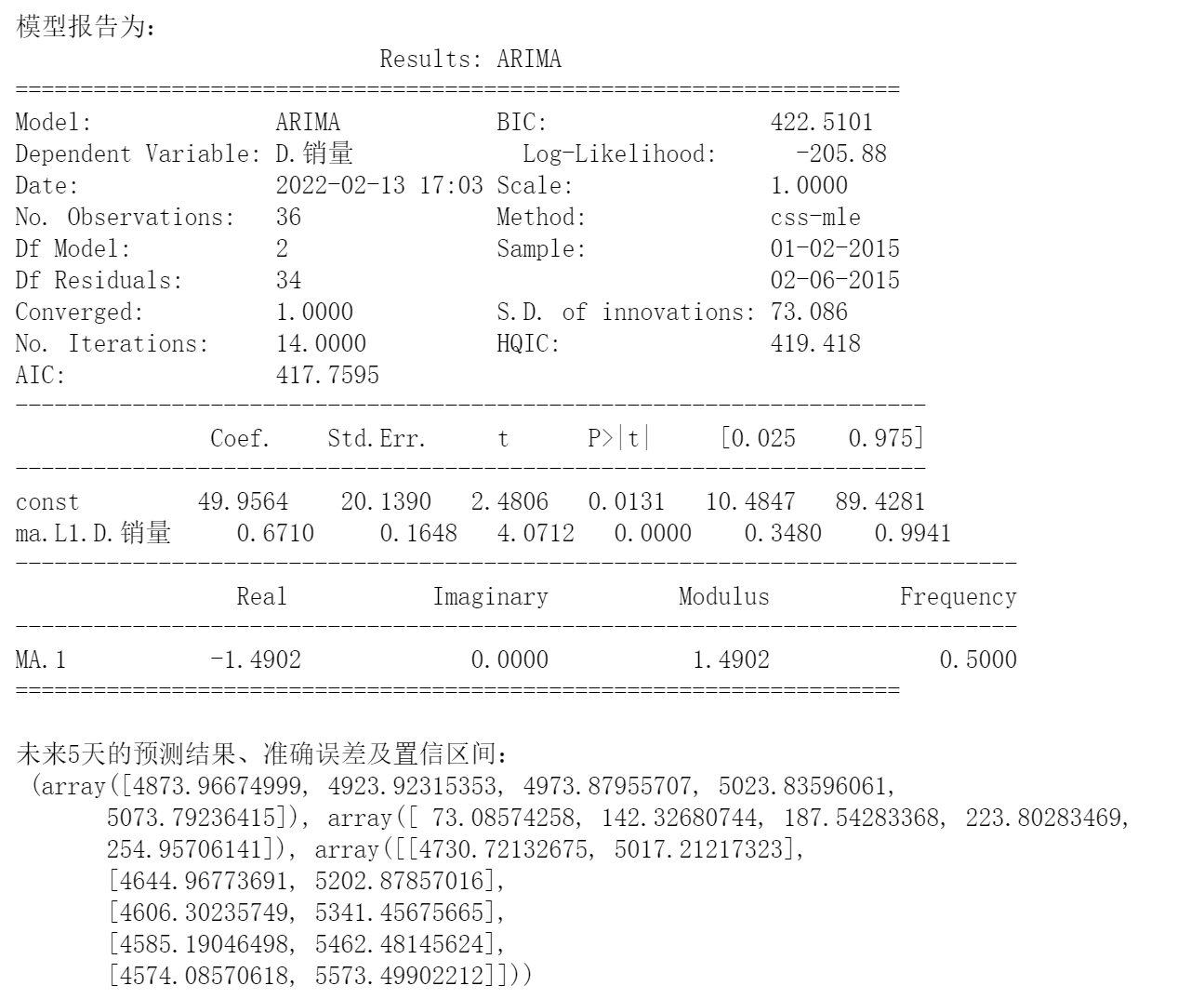展开全文机器学习 人工智能 深度学习
• 一、什么是时间序列? 时间序列是在规律性时间间隔记录的观测值序列。依赖于观测值的频率，典型的时间序列可分为每小时、每天、每周、每月、每季度和每年为单位记录。 import pandas as pd df = pd.read_csv('...

## 一、什么是时间序列?

时间序列是在规律性时间间隔记录的观测值序列。依赖于观测值的频率，典型的时间序列可分为每小时、每天、每周、每月、每季度和每年为单位记录。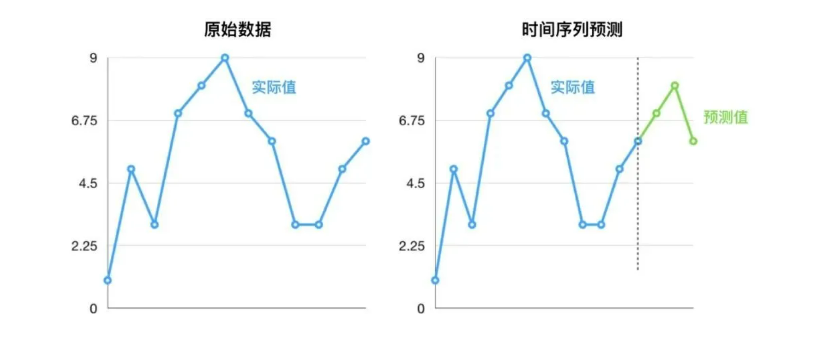import pandas as pd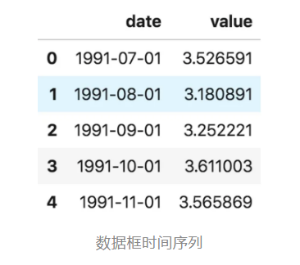# 二、时间序列具有4个成分，如下所示

• Level − It is the mean value around which the series varies.
• 水平 -它是序列变化的平均值。
• Trend − It is the increasing or decreasing behavior of a variable with time.
• **趋势 -**它是变量随时间的增加或减少行为。
• Seasonality − It is the cyclic behavior of time series.
• **季节性 -**这是时间序列的周期性行为。
• Noise − It is the error in the observations added due to environmental factors.
• 噪声 -这是由于环境因素导致的观测值误差。

## 三、预测质量评价指标

R 平方: 可决系数，取值范围为 [0,+∞)[0,+∞) ，其值越大，表示拟合效果越好 调用接口为 sklearn.metrics.r2_score，计算公式如下：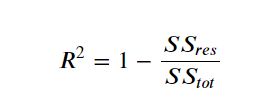平均绝对误差: 即所有单个观测值与算术平均值的偏差的绝对值的平均。这是一个可解释的指标，因为它与初始系列具有相同的计量单位。取值范围为 [0,+∞)[0,+∞) ，调用接口为 sklearn.metrics.mean_absolute_error ，计算公式如下：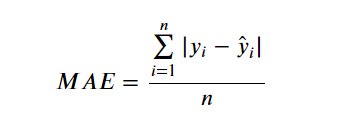中值绝对误差: 与平均绝对误差类似，即所有单个观测值与算术平均值的偏差的绝对值的中值。而且它对异常值是不敏感。取值范围为 [0,+∞)[0,+∞) ，调用接口为 sklearn.metrics.median_absolute_error ,计算公式如下：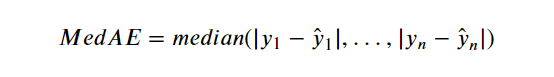**均方差：**最常用的度量标准，对大偏差给予较高的惩罚，反之亦然，取值范围为 [0,+∞)[0,+∞) ，调用接口为 sklearn.metrics.mean_squared_error ,计算公式如下：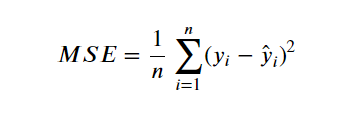均方对数误差: 这个与均方差类似，通过对均方差取对数而得到。因此，该评价指标也更重视小偏差。这指标通常用在呈指数趋势的数据。取值范围为 [0,+∞)[0,+∞) ，调用接口为 sklearn.metrics.mean_squared_log_error ,计算公式如下：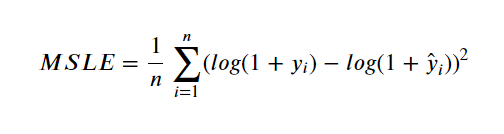**平均绝对百分比误差：**这与 MAE 相同，但是是以百分比计算的。取值范围为 [0,+∞)[0,+∞) ，计算公式如下：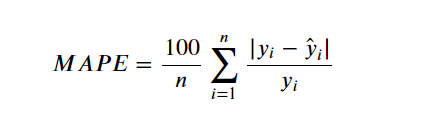#平均绝对百分比误差的实现
def mean_absolute_percentage_error(y_true, y_pred):
return np.mean(np.abs((y_true - y_pred) / y_true)) * 100


## 四、十大时序模型：

• Naïve, SNaïve
• Seasonal decomposition (+ any model)
• Exponential smoothing
• ARIMA, SARIMA
• GARCH
• Dynamic linear models
• TBATS
• Prophet
• NNETAR
• LSTM

1、Naïve,模型
我们依据过往的观测值来预测：

这类预测假设随机模型产出时间序列是一个random walk。

Naïve模型的扩展是SNaïve，SNaïve假设时间序列是有周期性的，而且其周期为T，则：

因此，以下T时间步的预测值与之前T时间步的预测值相等。Naïve和SNaïve模型通常被用作基准模型。

2、Seasonal decomposition (+ any model)
statsmodels是一个 Python 模块，它提供了用于估计许多不同统计模型以及进行统计测试和统计数据探索的类和函数。

安装：pip install statsmodels

如果数据显示出某种周期性（例如，每日、每周、每季度、每年），则我们可以将原始时间序列分解为三个部分的总和：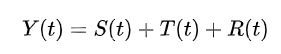其中S(t)是周期性部分，T(t)是趋势性成分，R(t)是剩余部分。针对这种分解，我们有多种经典的分解策略，例如：

• 我们可以通过rolling mean的策略来预估；
• 对于每个周期，我们通过计算平均去趋势序列来计算;
• 最后通过减法得到余数部分。

当然上面的策略还是相对简单，后来被优化为：

• 我们有非常数的周期；
• 通过分解的形式计算初始和最后的值；
• 防止过度平滑；
statsmodels.tsa.seasonal.seasonal_decompose（x，model = 'additive'，filt = None，period = None，two_side = True，extrapolate_trend = 0） #使用移动平均线进行季节性分解

参数：

x：array_like，被分解的数据

filt：tarray_like, optional，用于滤除季节性成分的滤除系数。滤波中使用的具体移动平均法由two_side确定

period：int, optional，系列的时期。如果x不是pandas对象或x的索引没有频率，则必须使用。如果x是具有时间序列索引的pandas对象，则覆盖x的默认周期性。

two_sided：bool, optional，滤波中使用的移动平均法。如果为True（默认），则使用filt计算居中的移动平均线。如果为False，则滤波器系数仅用于过去的值

extrapolate_trend：int or ‘freq’, optional，如果设置为> 0，则考虑到许多（+1）最接近的点，由卷积产生的趋势将在两端外推线性最小二乘法（如果two_side为False，则为单一个最小二乘）。如果设置为“频率”，请使用频率最近点。设置此参数将导致趋势或残油成分中没有NaN值。

# 下面深入分解：长期趋势Trend、季节性seasonality和随机残差residuals。

# 强行补充小知识：平稳性处理之“分解”
# 所谓分解就是将时序数据分离成不同的成分。statsmodels使用的X-11分解过程，它主要将时序数据分离成长期趋势、季节趋势和随机成分。

import statsmodels.api as sm  # 导入统计建模模块
# multiplicative
res = sm.tsa.seasonal_decompose(ts.values,freq=12,model="multiplicative")
# 这里用到的.tsa.seasonal_decompose()函数，经尝试：参数ts.values时，横坐标是Time；参数ts时，横坐标是date_block_num。其他不变。
# freg这个参数容后研究，这里暂且猜测是周期12个月。

# plt.figure(figsize=(16,12))
fig = res.plot()
# fig.show()  # 此句，可加可不加。

# 得到不同的分解成分，接下来可以使用时间序列模型对各个成分进行拟合。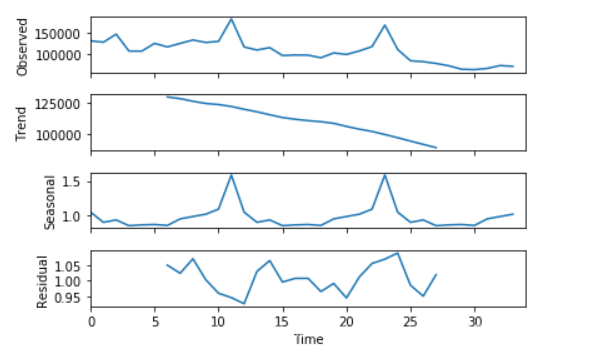3、指数平滑
指数平滑是早期大家在做数据竞赛时最为成功的一种模型，它基础形式为：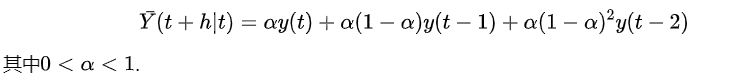使用指数平滑方法生成的预测是过去观测值的加权平均值，并且随着过去观测值离预测值距离的增大，权重呈指数型衰减。

权重减小率由平滑参数α控制。 如果α很大（即接近1），则对更近期的观察给予更多权重。 有两种极端情况：

1. α= 0：所有未来值的预测等于历史数据的平均值（或“平均值”），称为平均值法。

2. α= 1：简单地将所有预测设置为最后一次观测的值，统计中称为朴素方法。

Exponential smoothing：针对「没有趋势和季节性」的序列

1. 一次指数平滑，从最邻近到最早的数据点的权重呈现指数型下降的规律。

2. 当时间数列无明显的趋势变化，可用一次指数平滑预测。

Holt exponential smoothing：针对「有趋势但没有季节性」的序列

1. 二次指数平滑，通过引入一个额外的系数来解决指数平滑无法应用于具有趋势性数据的问题。

2. 二次指数平滑保留并更新两个量的状态：「平滑后的信号」和「平滑后的趋势」。

Holt-Winters exponential smoothing：针对「有趋势且有季节性」的序列

1. 三次指数平滑，通过再次引入一个新系数的方式同时解决了 Holt exponential smoothing
无法解决具有季节性变化数据的不足。

2. 三次指数平滑在此基础上引入季节性分量考虑时间序列周期性模式。

3. 有两种不同的季节性组成部分：

当季节变化在该时间序列中大致保持不变时，通常选择加法模型；

当季节变化与时间序列的水平成比例变化时，通常选择乘法模型；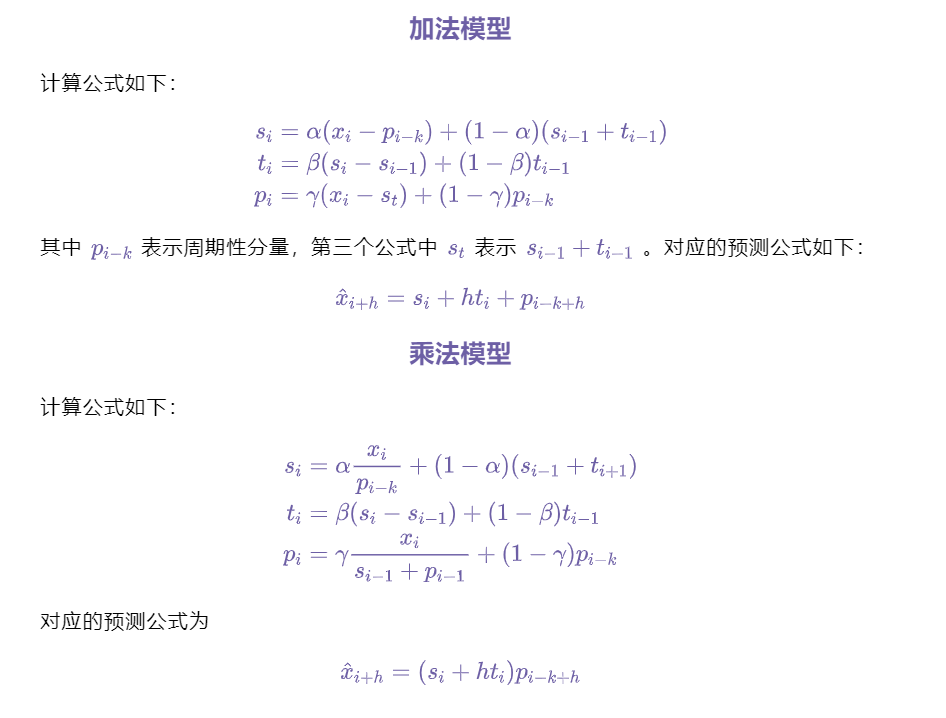import pandas as pd
import numpy as np
from scipy import  stats
import matplotlib.pyplot as plt
import statsmodels.api as sm

data.index=pd.Index(sm.tsa.datetools.dates_from_range('2020m1',length=len(data)))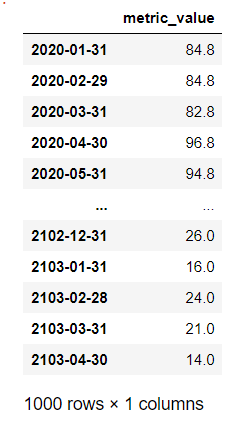from statsmodels.tsa.holtwinters import ExponentialSmoothing
model2=ExponentialSmoothing(data,seasonal_periods=12,trend='mul',seasonal='mul',freq='1M').fit()   #乘法模型
summary_1=model1.summary()
summary_2=model1.summary()
print(summary_1)
print(summary_2)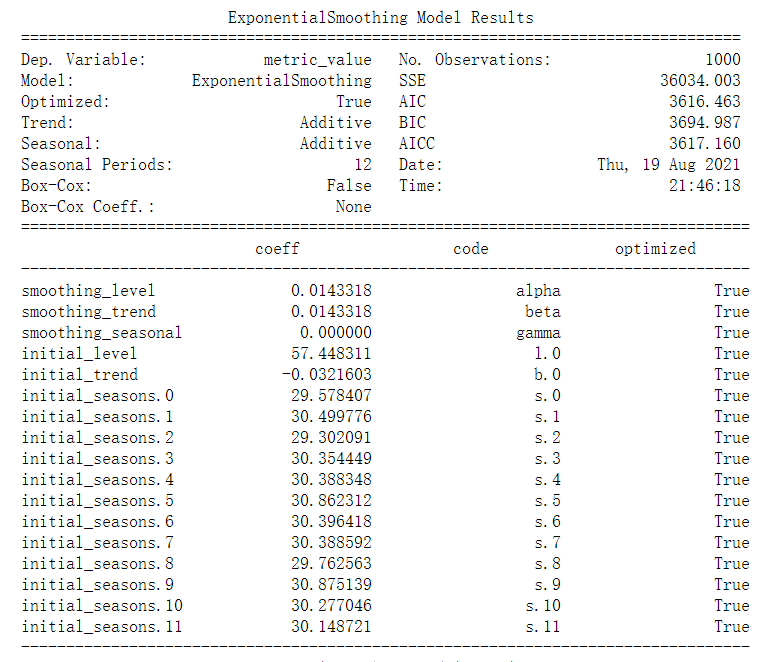#模型预测
model_forecast_2=model2.forecast(12)
print(model_forecast_2)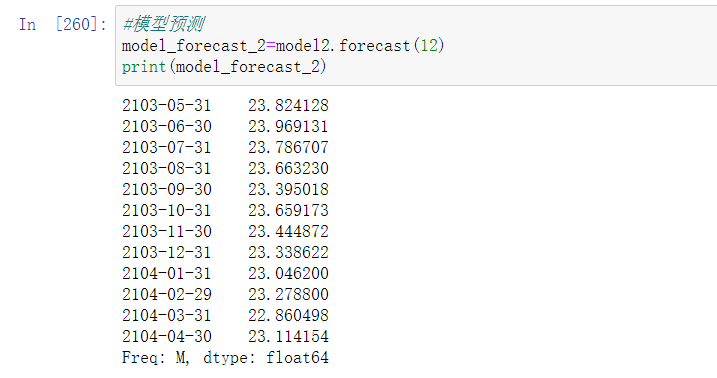展开全文sklearn 机器学习 人工智能 数据挖掘
• 时间序列模型简介   尽管此前我们已经用到了time series这个专业名词，但我们对时序特征进行的处理，并不是time series这个专业名词所代表的真正含义，既时间序列。尽管本阶段我们并不会讲解时间序列模型，但既然...

### 时间序列模型简介

尽管此前我们已经用到了time series这个专业名词，但我们对时序特征进行的处理，并不是time series这个专业名词所代表的真正含义，既时间序列。尽管本阶段我们并不会讲解时间序列模型，但既然讨论了时序特征，对时间序列略加了解，也是能够增加对时序特征处理方法理解的。因此以下部分作为补充内容，简单介绍下到底什么是时间序列模型。

注，此处仅对时间序列分析模型进行简单介绍，而时间序列分析模型本身也只是针对时序数据进行分析的诸多模型中的一种，其他能够进行时序规律挖掘的模型还包括RNN、LSTM等。

• 什么是时间序列模型

首先时间序列模型是一类拥有非常强统计背景的模型，尽管也属于有监督学习范畴，但其建模过程和机器学习的有监督学习算法大相径庭。时间序列是一种用于进行回归问题建模的模型，整个建模过程只有时间这一个特征，也就是说时间序列的模型预测过程就是希望通过时间这一个特征去对标签进行连续型数值预测

• 时间序列模型的建模过程

为了更好的理解时间序列模型，我们通过一组简单的例子来展示时间序列模型的一般建模过程。首先假设有如下数据集，特征只有月份这一个时间，标签是销售额，具体数值如下所示：对于上述数据集，特征和标签就不是简单的相关关系了。通过观察我们不难看出，标签的取值呈周期性变化，并且如果我们进一步将月份按照自然周期进行季度的划分，则会发现有如下规律：即销售额不再和月份相关，而是和每个季度的第几个月呈现出相关关系，那么也就是说，如果是围绕上述数据集进行预测，我们只需要将自然周期的月按照季度来进行划分，然后创建“季度中的第几个月”字段，即可利用该字段对销售额进行预测。当然，这种相关性还有另一种能够更加深刻的描述其本质的概念：自相关。
所谓自相关，指的是标签自己和自己相关，并且指的是某个时间点的自己和之前一段时间的自己是相关的。例如在上述数据集中，每个标签的取值，其实就和一个季度前的自己有极大的相关性（甚至取值都是一样的）：而标签一定要在时间维度上呈现出一定的自相关性，才能进一步带入时间序列模型进行建模预测。此外，这里的三个月时间差，也被称为按月计算的三阶差分，这里的销售额受到季节影响，也被称为销售额呈现季节性波动。

此处介绍的自相关性并不是自相关性概念的严谨定义、而自相关的概念也不仅仅出现在时间序列技术范畴内，望知悉。

当然，类似上述例举的，完全的自相关的情况还是比较少，更常见的情况是存在自相关、但也并不是完全自相关，例如如下数据集情况：能够看出，该数据集的标签在时间特征上仍然呈现出一定的自相关性，但这种相关性只存在于一个季度内，“三个月前的自己”和“现在的自己”都呈现出季度内稳步增长的情况，但也只是变化的趋势相同，Q2、Q1季度之间每月都相差1，而Q3和Q2之间每月则相差2，Q4和Q3之间每月相差3，哪怕间隔时间相同，随着时间变化，“过去的自己”和“现在的自己”差异也在逐渐增加，这种变化，也被称为时间序列中的趋势。对应到当前数据中来，就是整体序列的变化趋势是稳步增加的，即销售额尽管受到不同季度的季度周期影响，但整体是呈现上涨趋势的。
而时间序列中存在一些“趋势”，也是非常常见的一种情况。当然，除了一些季节性波动、长期趋势以外，有些时候还会出现一些偶然因素导致的时间序列发生变化，例如数据集如下所示：首先，该数据的销售额仍然存在季节性波动，同时呈现整体上涨的趋势，但其中6月、11月两个月的销售额稍显异常，通过和之前的数据集对比不难发现，该数据集的6月、11月销售额都增长了6万，这很可能是商家在6月和11月分别举办了“618”和“双11”促销活动所导致的销售额增加，而这些事件，也被称为不确定事件（并不是通过自然周期划分所决定的），往往在进行时间序列预测时，我们都需要把这些对时间序列有明显影响的随机事件找出来，合理评估其对标签的影响，然后利用再对预测的时间序列进行修正。
此外，在某些长期的预测项目中，还有可能出现一些循环的变动，例如某些行业受到经济周期影响，每个五到十年就会进行类似循环的变化，这也就是所谓的时间序列中的循环趋势。而对于时间序列模型来说，其根本作用就是去捕捉目标变量（标签）在时间维度中所呈现出来的季节波动、长期规律以及循环规律，然后再使用一些随机事件对标签取值进行修正，最后得出预测结果。当然，判断某数据集是否适合进行时间序列的建模，首先我们需要对其进行自相关性（以及偏自相关性）检验

从另一个角度来说，时序特征的周期划分就是捕捉周期性、关键时间差值就是趋势性与不规则规律挖掘。

• 时间序列模型本身的有效性

最后，我们来简单讨论时间序列模型本身的有效性。长期钻研机器学习的小伙伴肯定对只需要时间维度、这么一丁点数据就可以完成对标签数值预测的算法怀有迟疑态度，但实际上在我们的生产生活中，是存在很大一部分预测场景、能用且只能用时间序列模型来进行预测的。例如（很多小伙伴感兴趣的）股票预测，以及我个人曾经主导的四川省卫计委区域患病人次预测项目，也就是预测某地区的患病总人次。
在这些预测场景中，我们很难采用一般的机器学习模型进行建模，其中最难的地方在于无法有效的提取特征。对于区域患病人次预测项目来说，影响一个地区患病总人数的有效特征是非常难以提取及评估的，患病总人次可能和当地卫生状况、人口结构、气候、出行、节假日等诸多因素有关，而这些特征，要么受到随机事件影响根本无法提取（如大规模出行所导致的交叉感染）、要么根本无法量化评估（如卫生状况），而如果要通过气候变化对未来的一周甚至数周的患病人次进行预测，则还要依赖高度准确的天气预测结果。因此通过提取特征的方法进行机器学习预测，可以说是难上加难。
而另一方面，尽管区域患病总人次会受到诸多不确定因素影响，但在一个较长的时间段中，整体患病人次在时间维度上的分布还是有一定规律的，如下所示：（下图为2013-2016年四川省某地区14岁以下非特慢疾病接诊人次序列）据此，我们可以进一步对其进行自相关性检测、时间序列建模、随机事件修正等，最终模型的实时预测（按月）平均误差率仅在5%以内，可以说是非常高精度的预测结果了。

• 时间序列的模型局限

当然，时间序列本身的局限也非常明显，或者说时间序列本身就是一种只适用于谋者特殊情况的模型，即当一系列的不确定性因素产生了某种综合性的确定性的影响之后，时间序列能够非常快速的对其进行规律挖掘，但如果预测项目可以有效提取特征，那么机器学习模型肯定是潜力更大的模型。同样是患病人次预测，谷歌通过借助搜索引擎的搜索结果来进行的全美冬季流感人数预测，就会更加先进、更加高效、更加精准。此外，如果标签并未在时间维度上呈现周期性波动、趋势性变化，也没有自相关性，那么时间序列模型也是无法构建的。当然，哪怕是数据分布规律符合时间序列模型建模要求，时间序列的预测周期也会极大受到既有数据时间跨度影响，例如我们要预测接下来一个季度每个月的患病人次，那么可能就需要有过去两年的历史数据的支持，而如果要预测半年的患病人次，则需要有至少3-4年的历史数据支持，而在很多信息化建设较晚的领域，如此时间跨度的历史数据的获取，本身可能也是不小的难度。展开全文• ## 数学建模之时间序列模型及其应用

万次阅读 多人点赞 2020-08-12 21:38:42
时间序列模型就是将预测对象按照时间顺序排列起来,用这一组时间序列过去的变化规律,推断今后变化的可能性及变化趋势、变化规律。 时间序列模型也是一种回归模型,其一方面承认事物发展的延续性,运用过去的数据来推测...机器学习 MATLAB
• 第五章 时间序列模型的分析 第一节 时间序列模型简介 时间序列模型(time series model) 第二节 平稳时间序列模型 时序图 平稳时间序列的意义 例 5-1 白噪声时间序列(White noise time series) 一移动平均过程(MA ) ...
• 深度学习——时间序列模型评价指标总结_水龙吟唱的博客-CSDN博客_时间序列预测评价指标 机器学习中的预测评价指标MSE、RMSE、MAE、MAPE、SMAPE_府学路18号车神的博客-CSDN博客_预测评价指标时间序列预测的常见方法...
• ## 常见时间序列模型

万次阅读 2018-12-30 11:50:22
本文主要对各种时间序列模型及其特征做了一个归纳总结，以便查询了解。 符号说明： 变量： x,yx,yx,y 变量集：X,YX,YX,Y 变量xxx在ttt时刻的值：xtx_txt​ 参数：α,β\alpha, \betaα,β ##自回归模型...VAR
• 1. 时间序列规则 1.1 什么是时间序列规则 对于赛题/业务的规则之前已经描述了它的重要性和应用，在此不再赘述。这章主要了解时间序列及其规则，和周期的应用。 1.1.1 时间序列 时间序列（或称动态数列）：指将同一...
•python
• 时间序列模型一、获取数据源二、缺失值处理三、检验序列的稳定性四、序列平稳化五、参数寻优六、建立模型七、模型检验八、模型预测 美股封盘(close)数据 获取数据源—>缺失值处理—>检验数据稳定性—>序列...数据挖掘 机器学习 python
• 时间序列的分析的步骤是先对数据进行平稳性和非白噪声检验（如不满足需对数据进行平滑或差分等预处理），然后才是模型调参跟预测，因此本文分为2大部分介绍，答题思路见脑图。 一、数据准备&探索 1、平稳性 ...python
• 原始数据可能并不是时间序列，因此，对这样的数据建立时间序列模型，分为以下几步： 1 生成时间序列：定义新的时间变量，并对原始数据进行平稳处理 a)定义时间变量： 选择按什么时间序列进行定义： b)平稳...
• ## 算法模型---时间序列模型

万次阅读 多人点赞 2018-01-16 09:04:58
1、时间序列时间序列是时间间隔不变的情况下收集的不同时间点数据集合，这些集合被分析用来了解长期发展趋势及为了预测未来。...常用的时间序列模型有AR模型、MA模型、ARMA模型和ARIMA模型等。2、时间数据
• ## 时间序列模型 （一）：模型概述

万次阅读 多人点赞 2019-04-21 21:47:12
时间序列的其它博文系列： 时间序列模型 （一）：模型概述...时间序列模型 （六）：平稳时间序列模型 ：自回归AR 、移动平均 MA 、ARMA 模型 时间序列模型 （七）： 时间序列建模的基本步骤 目录 时间序列的不同...
• 一、时间序列概念 在生产和科学研究中，对某一个或一组变量x(t)进行观察测量，将在一系列时刻t1, t2, …, tn (t为自变量)按照时间次序排列，并用于解释变量和相互关系的数学表达式。在相等的时间间隔内收集到的不同...
• 本文主要介绍了时间序列的平稳性、AR、MA、ARMA等内容
• 时间序列模型 - Prophet1.时间序列简介1.1 时间序列 - 平稳性检验1.1.1 log法1.1.2 差分法1.2 平稳性的单位根检验2.ARIMA3.Prophet3.1 Prophet的优点3.2 安装Prophet3.3 数据处理3.4 预测4.LSTM4.1 数据处理4.2 训练...python
• SPSS(十九)SPSS之时间序列模型（图文+数据集） 时间序列是指将同一统计指标的数值按其发生的时间先后顺序排列而成的数列。正如人们常说，人生的出场顺序很重要，时间序列中隐藏着一些过去与未来的关系。时间序列...spss时间序列预测 自相关偏自相关
•人工智能 自然语言处理 nlp HMM CRF
• 而复杂的模型，还是有可能把正确率提升1个百分点以上的 ，否则quant干什么吃呢？ 7 甚至有些初看觉得特别有规律的东西， 也不是完全可预测的 比如心跳这个看似简单的问题， 首先心跳是个时间序列，然后心跳不是...
• 时间序列预测首先要确定预测的内容。 本文预测共享单车的日租借量 使用的是每日数据 预测的时间范围是需要提前一个月、半年还是一年？根据预测范围，会使用到不同的模型。 首先安装加载本文所需要的包 install....机器学习 r语言
• 对于采用的每个统计/机器学习（ML）模型， 都使用默认超参数。当然，进一步调整它们各自的超参数可能会获得更好的预测性能。 本文来自《数据黑客》，登录官网可阅读更多精彩资讯和文章。 1. 单变量时间序列 Nile数据...机器学习 r语言 大数据...